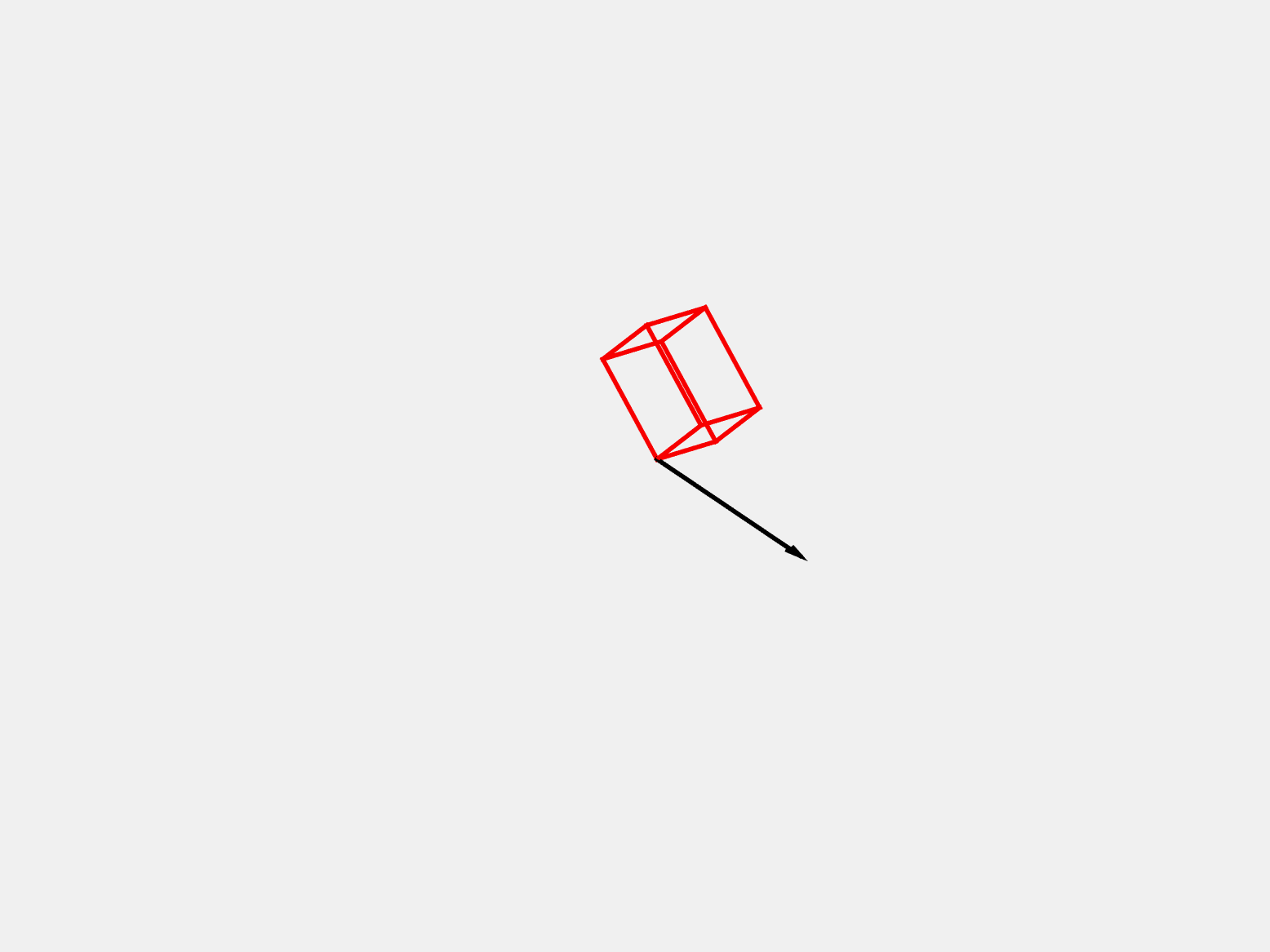#### Type I TwinsType I twins are most easily visualized through a reflection across a plane called the twin plane. In this animation, the unit cell (or deformation gradient) to the left of the twin plane is reflected across the twin plane. This results in a slightly different unit cell (or deformation gradient). The two unit cells (or deformation gradients) are symmetry-related correspondence variants of each other. Then, the two variants are populated on each side of the twin plane. When the resulting structure is rotated, you can see the familiar "tent" or "kink" often seen in twinned microstructures.

#### Type II TwinsType II twins are most easily visualized by a rotation around a vector called the shear vector. In this animation, the red unit cell (or deformation gradient) is rotated around the shear vector to create the blue unit cell (or deformation gradient). This results in a slightly different unit cell (or deformation gradient). The two unit cells (or deformation gradients) are symmetry-related correspondence variants of each other. Then, the two variants are populated on each side of the twin plane.

#### Compound Twins

Compound twins can be easily visualized either through a reflection across the twin plane or through a rotation around the shear vector.